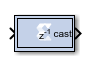# Vector Convert - 2020.2 English

## Vivado Design Suite Reference Guide: Model-Based DSP Design Using System Generator (UG958)

Document ID
UG958
Release Date
2020-11-18
Version
2020.2 English

The Vector Convert block supports Data Type Conversion feature for vector type inputs.

Hardware notes: Rounding and saturating require hardware resources; truncating and wrapping do not.

## Description

Super Sample Rate (SSR): This configurable GUI parameter is primarily used to control processing of multiple data samples on every sample period. This blocks enable 1-D vector data support for the primary block operation.

The Vector Convert block converts each input sample to a number of a desired arithmetic type. For example, a number can be converted to a signed (two's complement) or unsigned value.## Block Parameters

Open the Block Parameters dialog box by double-clicking the icon in your Simulink® model.

Basic tab

Parameters specific to the Basic Tab are as follows:

Output Type
• Specify the output data type.
• Boolean
• Fixed-point
• Floating-point
Arithmetic Type
If the Output Type is specified as fixed-point, you can select Signed (two’s comp) or Unsigned.
Fixed-point Precision
Number of bits
Specifies the bit location of the binary point, where bit zero is the least significant bit
Binary point
Specifies the bit location of the binary point, where bit zero is the least significant bit.
Floating-point Precision
Single
Specifies single precision (32 bits).
Double
Specifies double precision (64 bits).
Custom
Activates the field below so you can specify the Exponent width and the Fraction width.
Exponent width
Specify the exponent width.
Fraction width
Specify the fraction width.
Quantization

Quantization errors occur when the number of fractional bits is insufficient to represent the fractional portion of a value. The options are to Truncate (for example, to discard bits to the right of the least significant representable bit), or to Round(unbiased: +/- inf) or Round (unbiased: even values).

Round(unbiased: +/- inf) also known as "Symmetric Round (towards +/- inf)" or "Symmetric Round (away from zero)". This is similar to the MATLAB `round()` function. This method rounds the value to the nearest desired bit away from zero and when there is a value at the midpoint between two possible rounded values, the one with the larger magnitude is selected. For example, to round 01.0110 to a Fix_4_2, this yields 01.10, since 01.0110 is exactly between 01.01 and 01.10 and the latter is further from zero.

Round (unbiased: even values) also known as "Convergent Round (toward even)" or "Unbiased Rounding". Symmetric rounding is biased because it rounds all ambiguous midpoints away from zero which means the average magnitude of the rounded results is larger than the average magnitude of the raw results. Convergent rounding removes this by alternating between a symmetric round toward zero and symmetric round away from zero. That is, midpoints are rounded toward the nearest even number. For example, to round 01.0110 to a Fix_4_2, this yields 01.10, since 01.0110 is exactly between 01.01 and 01.10 and the latter is even. To round 01.1010 to a Fix_4_2, this yields 01.10, since 01.1010 is exactly between 01.10 and 01.11 and the former is even.

Overflow

Overflow errors occur when a value lies outside the representable range. For overflow the options are to Saturate to the largest positive/smallest negative value, to Wrap (for example, to discard bits to the left of the most significant representable bit), or to Flag as error (an overflow as a Simulink error) during simulation. Flag as error is a simulation only feature. The hardware generated is the same as when Wrap is selected.

Optional Ports

Provide enable port: Activates an optional enable (en) pin on the block. When the enable signal is not asserted the block holds its current state until the enable signal is asserted again or the reset signal is asserted.

Latency

The Latency value defines the number of sample periods by which the block's output is delayed. One sample period might correspond to multiple clock cycles in the corresponding FPGA implementation (for example, when the hardware is over-clocked with respect to the Simulink model). System Generator will not perform extensive pipelining unless you select the Pipeline for maximum performance option (described below); additional latency is usually implemented as a shift register on the output of the block.

Implementation tab

Parameters specific to the Implementation tab are as follows:

Performance Parameters
Pipeline for maximum performance

The Xilinx LogiCORE™ can be internally pipelined to optimize for speed instead of area. Selecting this option puts all user defined latency into the core until the maximum allowable latency is reached. If the Pipeline for maximum performance option is not selected and latency is greater than zero, a single output register is put in the core and additional latency is added on the output of the core.

The Pipeline for maximum performance option adds the pipeline registers throughout the block, so that the latency is distributed, instead of adding it only at the end. This helps to meet tight timing constraints in the design.

Other parameters used by this block are explained in the topic Common Options in Block Parameter Dialog Boxes.